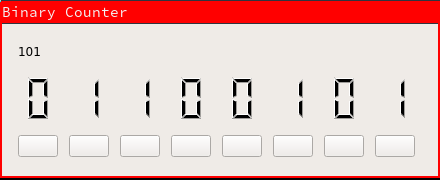# danieljon.es

index posts opinions likes dislikes interesting cgit

# Posts

My posts about programming and things.
Date format is day/month/year because I'm sane.

# Binary

### 6/8/2017

Surprisingly i've never looked at learning how to count in binary, so, the other night I did.

Binary is a base 2 counting system, meaning you have 2 numbers available, 0 and 1. Most humans use base 10 (give this some research, interesting stuff), the numbers 0 through to 9 and considering we've grown up with thsi technique, it's easy to us. I was surprised at how simple it is to count in binary utilising your own base 10 knowledge.

For this post we're going to be using 8 bits (which equates to one byte, better known as a single character like 't'). A bit is a single 0 or 1, so 8 bits equates to something like this '0 0 1 0 1 1 0 0' which is the number 44.

But, how do those seemingly random 0's and 1's equal 44? This is where things get fun.

each of those bits (8 either 0's or 1's) equates to a number, this number is doubled for every bit, for example, using 4 bits the value increases like '1, 2, 4, 8). However, in binary these numbers are REVERSED '8, 4, 2, 1', the most significant number on the left.

Let's set our our 8 bits in order to equate 44:
128 64 32 16 8 4 2 1
Now, let's place our 0's and 1's in their correct position:

128
64 32 16 8 4 2 1
0 0 1 0 1 1 0 0
Now, for each column with a 1, add them together:
32 + 8 + 4 = 44. This is the binary representation of 44.
With these 8 bits we can count from 0 to 255 (entire ASCII table), awesome.

So with this knowledge and a little practice you can begin to count using binary pretty easily. But, now you want to spell out words, well, you can do that too.

ASCII is a character encoding standard that is utilised in computers to convert decimals (previously binary) into characters us humans can understand. Using an ASCII table we can do this conversion ourself manually.

Lets utilise the lowercase english alphabet, as shown in the ASCII table the lower case 'a' is represented by the number 97 and the lowercase 'z' being 122, filling in the gaps we have the entire alphabet. Take note that the uppercase alphabet is represented from 65 to 90. Knowing this, writing out our name for example using binary is extremely easy, however quite time consuming. Let's do my name, 'daniel'.
01100100 = 100 = d
01100001 = 97 = a
01101110 = 110 = n
01101001 = 105 = i
01100101 = 101 = e
01101100 = 108 = l
Let's plug these numbers into an online binary to text converter such as this free website and input the string "01100100 01100001 01101110 01101001 01100101 01101100". Pretty simple in the end.

You can also utilise a neat trick designed to allow converting binary to text quickly. Take the last 5 bits of data ignoring the first 3, for example '01100' from the character 'l' above. Add the rows with 1's together, in this case 8 and 4 which equals 12. Now what is the 12th letter of the alphabet? That's right, 'l'. This trick works for both upper and lowercase characters, the difference being the first 3 bits of a lowercase character are 011, while for uppercase characters it is 010.

I created an 8-bit binary counter using Qt and C++ in order to practice my counting, it provides a real-time calculation of your number. You can find the source code here.page generated 16-03-2019 13:35:17 using sitegenerator